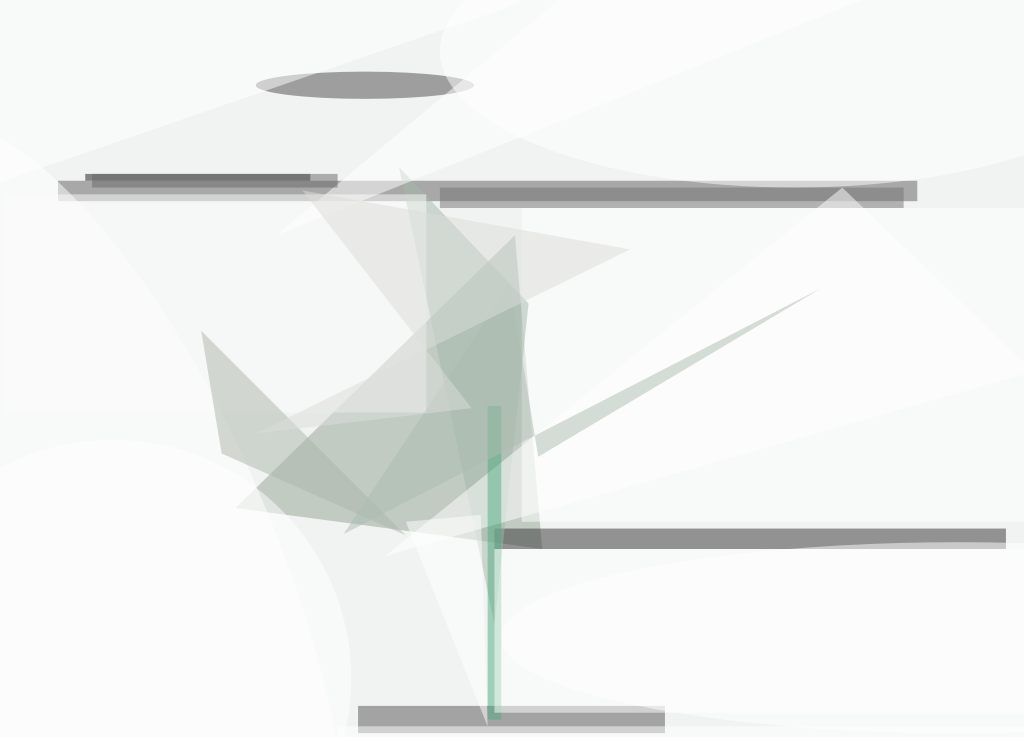A high-power laser beam can be used to apply a torque to a sample of material that is electromagnetically and/or electrostatically levitated in a vacuum. The torque can be used to alter the state of rotation of the sample; this is an important capability because control of the state of rotation (or lack of rotation) can be necessary for processing the sample and/or measuring its properties.The Laser Beam Impinges on the Sample off center. The torque imparted to the sample is proportional to the fraction of the beam power absorbed and to the length, a, of the moment arm.

In the usual case of an approximately spherically symmetrical sample, torque is generated by simply aiming the laser beam off center (see figure). It does not matter whether the sample is solid or molten, nor does it matter whether the sample is electrically conductive or nonconductive; this is because the torque-generating mechanism is simply the transfer of momentum to the sample from photons that impinge on the sample along a line that does not pass through the center of mass of the sample. The magnitude of the torque depends on the power of the laser beam, the fraction of incident photons absorbed (reflected photons do not contribute to torque), and the size of the moment arm.

The magnitude of the torque can readily be estimated for the case in which the laser used to apply the torque to a spherical sample of massm and radius R is the same laser used to heat the sample to a steady-state temperature, T. Assuming that the absorption and emission of radiation by the sample is governed by the Stefan-Boltzmann law, the torque (t ) is given by

τ = 4 πR2 σ e aT4/c,

where s is the Stefan-Boltzmann constant, e is the hemispherical total emissivity of the sample material, a is the length of the moment arm, and c is the speed of light. The angular acceleration (f') of the sample in this case is given by

This work was done by Won-Kyu Rhim and Paul-François Paradis of Caltech for NASA's Jet Propulsion Laboratory.

NPO-20731

##### This Brief includes a Technical Support Package (TSP).###### Laser-Induced Rotation of a Levitated Sample in a Vacuum